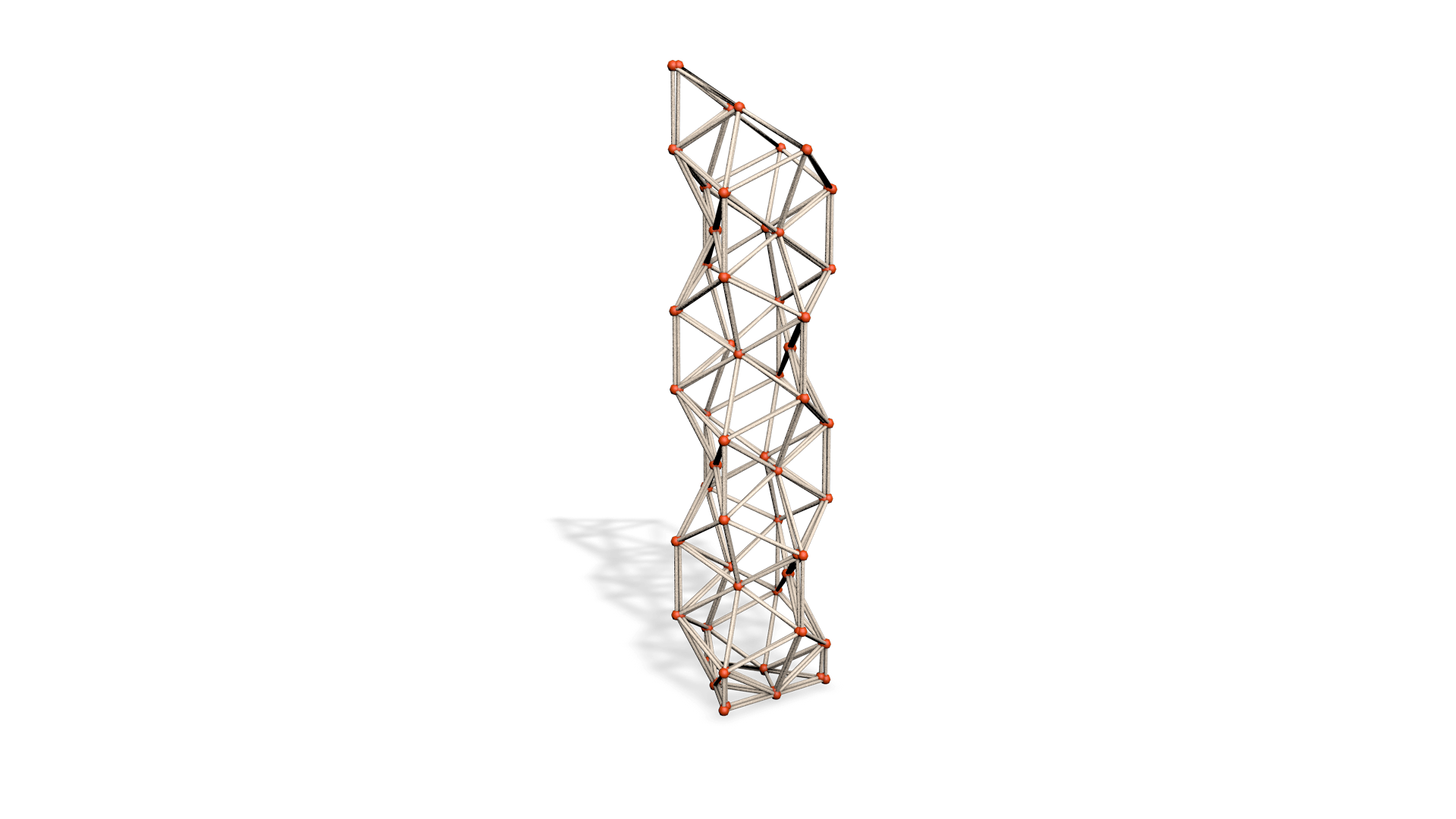# CC / Form follows function

Attempt to solve the problem of exploring all possible build-able shapes from a given set of various triangles.

Defining

• the joint: a point in 3D space
• joint matrix: a list of all joints in the scene
• shape matrix: a matrix of boolean values with columns and rows equal to the total number of joints, storing a true value where two joints are connected and false otherwise.
• craft elements: a joint matrix and a shape matrix describing sizes from real objects scansJoint Matrix:

1.    x,  y,  z
2.    1, 1.4, 1.2
3. 1.2, 1.1, 1.9
4. 1.4, 1.6, 1.1

Shape Matrix:

1 2 3 4 5 …

1.  F F T F F
2.  T F F F F
3.  F F F T F
4.  F F T F T
5.  T F F T F

Approaches

Potential use of Unity Engine’s triangulation of shapes for solving the way triangles can define any 3D shape. (Delaunay Triangulation could be implemented for more control over distribution.)

Applications:

• Creating the structure of real objects using real object 3D scans (available at this point only on Apple ARKit, announced on Niantic and requested on Google ARCore)
• Recreation of abstract objects with a minimal use of mathematics, relying on Unity’s Engine 3D calculations.Breakdown of shapes and implementing of algorithms to solve the spatial placement of the joints and triangles, potentially linking to machine learning.

Algorithm Candidates

• Backtracking (will be slowest but given small amount of data to process could turn out to be practical)
• Brench and bound (at first glance seems as a potential solution)
• Graph algorithms if the shape is reinterpreted to fit the algorithm.

Applications

• limitless freedom in the shape, here we have individual control over triangles and further more squared or and n-polygon shape that acts as main construction element.
• AI can truly propose the most efficient models that can be computer calculated because of it having access as well to every element in the shape.
• Remixing shapes while keeping them realistic.Give a quick feedback
+1
0
+1
0
+1
0
+1
0
+1
0
+1
0
+1
0